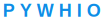# 8.1 用例故事### 8.1.1 应用故事1. 将地理化坐标[Xserial，Yserial] 相同的记录进行分组。

2. 将同一组内的多条记录的温度采样点数，低温采样点数进行聚合操作，聚合的算法是加法，大概的意涵是：

假设按照地理化坐标[Xserial，Yserial]分组后，每一组中有 N 条记录。

聚合（温度采样点数）=sum（温度采样点数 1+温度采样点数 2+… 温度采样点数 N）；

聚合（温度采样点数）= sum（低温采样点数 1+低温采样点数 2+… 低温采样点数 N）### 8.1.2 用例描述

1. 用户输入期待处理的文件路径，输出结果文件的，相关的信息结构

[ 输入文件绝对路径，输出文件绝对路径]

1. 程序读取 grid.xlsx 文件，基于 pandas 转换为内存中的 dataframe，成为一个 Data set 的格式，命名为 DF1。

2. 程序根据入参中“输出文件绝对路径”，创建一个输出结果 gropu-geo-grid.xlsx 文件的句柄。；

3. 应用程序对 Data set 的表头进行扩展，增加一列，名字分别为

1. 应用程序针对 Dataframe 中的 字段 X-Y 进行分组操作，并对字段“温度采样次数“和”低温采样点数“ 进行聚合操作，聚合的算法是 sum，就是将相关的数值进行相加，其中数值为整型数据。

1. 应用程序将内存中的 data set，也就是新生成的 DF2， 写入输出结果 xlsx 文件的句柄， 相关文件决对路径来自输入数据。

1. 应用程序返回程序执行响应 [ 执行结果，输出结果文件信息]

## 8.2 用例约束

1. python 使用 python3

2.文件读写使用 pandas 组件

3.数据操作使用 pandas 组件

## 8.3 设计方案简介

### 8.3.2 调用关系### 8.3.3 流穿越2.合并新字段

``````new_data["x-y"]= new_data["Xserial"].map(str)+"-"+new_data["Yserial"].map(str)
``````3、分组聚合操作

``````res=new_data.groupby(["Xserial","Yserial","x-y"]).agg({"温度采样次数":"sum","低温采样点数":"sum"})
``````1. 写入本地## 8.4 设计方案详解

### 8.4.1 领域对象模型1.向新生成的 DF 中，增加一个新的字段 X-Y。

2.根据字段 X-Y，进行分组聚合操作。

2、分组聚合操作数据分组，合并

### 8.4.3 字段拼接，df-str 属性

Df.str

#### 8.4.3.3 case 举例

##### 1 新建一个 df##### 2 方案一，通常方案 map 与 apply##### 3、 方案二，str 方法### 8.4.4 分组聚合操作

#### 8.4.4.1 应用目的

Information 提取，从众多 data 过滤所需的信息

#### 8.4.4.2 语法说明#### 8.4.4.3 case 举例

##### 1、分组聚合模型图##### 2、新建一个 df##### 3. 进行分组聚合##### 4、 自定义聚合函数##### 5、根据多个键进行分组和聚合### 8.4.5 使用 pandas 读/写 excle

#### 8.4.5.1 应用目的

Excel 是微软的经典之作，在日常工作中的数据整理、分析和可视化方面，有其独到的优势，尤其在你熟练应用了函数和数据透视等高级功能之后，Excel 可以大幅度提高你的工作效率。但如果数据量超大，Excel 的劣势也就随之而来，甚至因为内存溢出无法打开文件，后续的分析更是难上加难。那么，有什么更好的解决办法吗？工欲善其事，必先利其器，在这里我们介绍使用 Python 的 pandas 数据分析包来解决此问题。

Storage 阶段，data 的读取与 information 的存储。

#### 8.4.5.2 语法说明

``````pd.read_excel(io, sheet_name=0, header=0, names=None, index_col=None,

usecols=None, squeeze=False,dtype=None, engine=None,

converters=None, true_values=None, false_values=None,

skiprows=None, nrows=None, na_values=None, parse_dates=False,

date_parser=None, thousands=None, comment=None, skipfooter=0,

convert_float=True, **kwds)
``````

#### 8.4.5.3 case 举例

##### 1、io，Excel 的存储路径

``````import pandas as pd

``````
##### 2、sheet_name，要读取的工作表名称

``````data = pd.read_excel(io, sheet_name = 1)

``````

``````data = pd.read_excel(io, sheet_name = '英超射手榜')

``````SheetN：代表第 N 个 sheet，S 要大写，注意与整型数字的区别。

``````data = pd.read_excel(io, sheet_name = 'Sheet5')

``````sheet_name 默认为 0，取 Excel 第一个工作表。如果读取多个工作表，则显示表格的字典。对于初学者而言，建议每次读取一个工作表，然后进行二次整合。

``````data = pd.read_excel(io, sheet_name = ['英超积分榜', '西甲积分榜'], nrows = 5)

sheet_name = ['英超积分榜', '西甲积分榜'] ，返回两个工作表组成的字典
``````

data``````data = pd.read_excel(io, sheet_name = '英超积分榜', header = [0,1])
``````

# 前两行作为列名。

``````data.head()
``````##### 4、names， 自定义最终的列名

``````data = pd.read_excel(io, sheet_name = '英超射手榜',

names = ['rank','player','club','goal','common_goal','penalty'])

``````##### 5、index_col， 用作索引的列

``````data = pd.read_excel(io, sheet_name = '英超射手榜', index_col = '排名')

````````````data = pd.read_excel(io, sheet_name = '英超射手榜', index_col = [0, 1])

``````##### 6、usecols，需要读取哪些列

usecols 可避免读取全量数据，而是以分析需求为导向选择特定数据，可以大幅提高效率。

``````data = pd.read_excel(io, sheet_name = '西甲射手榜', usecols = [0, 1, 3])

````````````data = pd.read_excel(io, sheet_name = '西甲射手榜', usecols = 'A:C, E')

``````##### 7、squeeze，当数据仅包含一列

squeeze 为 True 时，返回 Series，反之返回 DataFrame。

``````data = pd.read_excel(io, sheet_name = 'squeeze', squeeze = True)

````````````data = pd.read_excel(io, sheet_name = 'squeeze', squeeze = False)

``````##### 8、converters ，强制规定列数据类型
``````converters = {'排名': str, '场次': int}， 将“排名”列数据类型强制规定为字符串（pandas默认将文本类的数据读取为整型），“场次”列强制规定为整型；
``````

``````data = pd.read_excel(io, sheet_name = 'converters')

data['排名'].dtype

data = pd.read_excel(io, sheet_name = 'converters', converters = {'排名': str, '场次': float})

data['排名'].dtype
``````##### 9、skiprows，跳过特定行

skiprows= n， 跳过前 n 行； skiprows = [a, b, c]，跳过第 a+1,b+1,c+1 行（索引从 0 开始）；

``````data = pd.read_excel(io, sheet_name = '英超射手榜', skiprows = [1,2,3])
``````

# 跳过第 2,3,4 行数据（索引从 0 开始，包括列名）

``````data.head()
````````````data = pd.read_excel(io, sheet_name = '英超射手榜', skiprows = 3)

``````##### 10、nrows ，需要读取的行数

``````data = pd.read_excel(io, sheet_name = '英超射手榜', nrows = 10)
``````##### 11、skipfooter ， 跳过末尾 n 行
``````data = pd.read_excel(r'C:\Users\Administrator\Desktop\data.xlsx' ,

sheet_name = '英超射手榜', skipfooter = 43)

# skipfooter = 43， 跳过末尾 43 行（索引从 0 开始）
``````1.4.5.4 注意事项

Excel 没有追加模式，可以用下图方式写入文件### 8.4.6 更改 df 一列的数据类型

#### 8.4.6.2 语法说明

df[“x 列”].map(str)

#### 8.4.6.3 case 举例Df 数据如下：Starter
MicroServ
Tutorials
Report
Blog